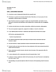coralserval833 and 79 others unlocked12
Verified Note
12 documents

## Document Summary

Topics covered : numeric values, variables, assignment, identifiers, reserved words. Integer values: example: number 1, integers are whole numbers, no fractions, can be positive , negative or zero, example: >>print( x = + str(x)) x = 100. X = 100: tuple assignment , example: x, y, z = 100 , -45, 0. >>>print( x = , x , y = , y, z = , z) X = 100 y = -45 z= 0. Naming and using variable: variables can be numbers, letters and underscores, spaces are not allowed in variable names, no using python keywords as variable names, name should be short and descriptive. A string is a series of characters. Anything inside single or double quotes is a string.

## Get access